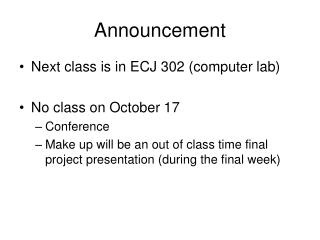Download PresentationAnnouncement

# Announcement - PowerPoint PPT Presentation

Announcement. Next class is in ECJ 302 (computer lab) No class on October 17 Conference Make up will be an out of class time final project presentation (during the final week). Lecture Objectives:. Analyze the unsteady-state heat transfer numerical calculation methods. Example:.I am the owner, or an agent authorized to act on behalf of the owner, of the copyrighted work described.
Download Presentation## Announcement

Download Policy: Content on the Website is provided to you AS IS for your information and personal use and may not be sold / licensed / shared on other websites without getting consent from its author.While downloading, if for some reason you are not able to download a presentation, the publisher may have deleted the file from their server.

- - - - - - - - - - - - - - - - - - - - - - - - - - E N D - - - - - - - - - - - - - - - - - - - - - - - - - -
Presentation Transcript
1. Announcement • Next class is in ECJ 302 (computer lab) • No class on October 17 • Conference • Make up will be an out of class time final project presentation (during the final week)

2. Lecture Objectives: • Analyze the unsteady-state heat transfer numerical calculation methods

3. Example: Unsteady-state heat transfer(Explicit – Implicit methods) To - known and changes in time Tw - unknown Ti - unknown Ai=Ao=6 m2 (mcp)i=648 J/K (mcp)w=9720 J/K Initial conditions: To = Tw = Ti = 20oC Boundary conditions: hi=ho=1.5 W/m2 Tw Ti To Ao=Ai Conservation of energy: Time step Dt=0.1 hour = 360 s

4. Conservation of energy equations: Explicit – Implicit methods example Wall: Air: After substitution: For which time step to solve: +  or  ? Wall: Air: +  Implicit method  Explicit method

5. Implicit methods - example After rearranging: 2 Equations with 2 unknowns!  =0 To Tw Ti  =36 system of equation Tw Ti  =72 system of equation Tw Ti

6. Explicit methods - example  =360 sec  =0 To Tw Ti  =360 To Tw Ti  =720 To Tw Ti Time There is NO system of equations! UNSTABILE

7. Explicit method Problems with stability !!! Often requires very small time steps

8. Explicit methods - example  =0 To Tw Ti  =36 To Tw Ti  =72 To Tw Ti Stable solution obtained by time step reduction 10 times smaller time step Time  =36 sec

9. Explicit methods information progressing during the calculation Tw Ti To

10. Unsteady-state conduction - Wall q Nodes for numerical calculation Dx

11. Discretization of a non-homogeneous wall structure Section considered in the following discussion Discretization in space Discretization in time

12. Internal node Finite volume method Surface node Boundaries of control volume For node “I” - integration through the control volume

13. Mathematical approach(finite volume method) Left side of equation for node “I” - Discretization in Time Right side of equation for node “I” - Discretization in Space

14. Mathematical approach(finite volume method) Using and for uniform grid By Substituting left and right side terms of equation we get the following equation for Explicit method Implicit method

15. Physical approach(finite volume method) qx Change of heat flux along x Change of energy in x = Boundaries of control volume or For finite volume x: Sum of energy that goes in and out of control volume x Change of energy in x =

16. Physical approach(finite volume method) For uniform grid